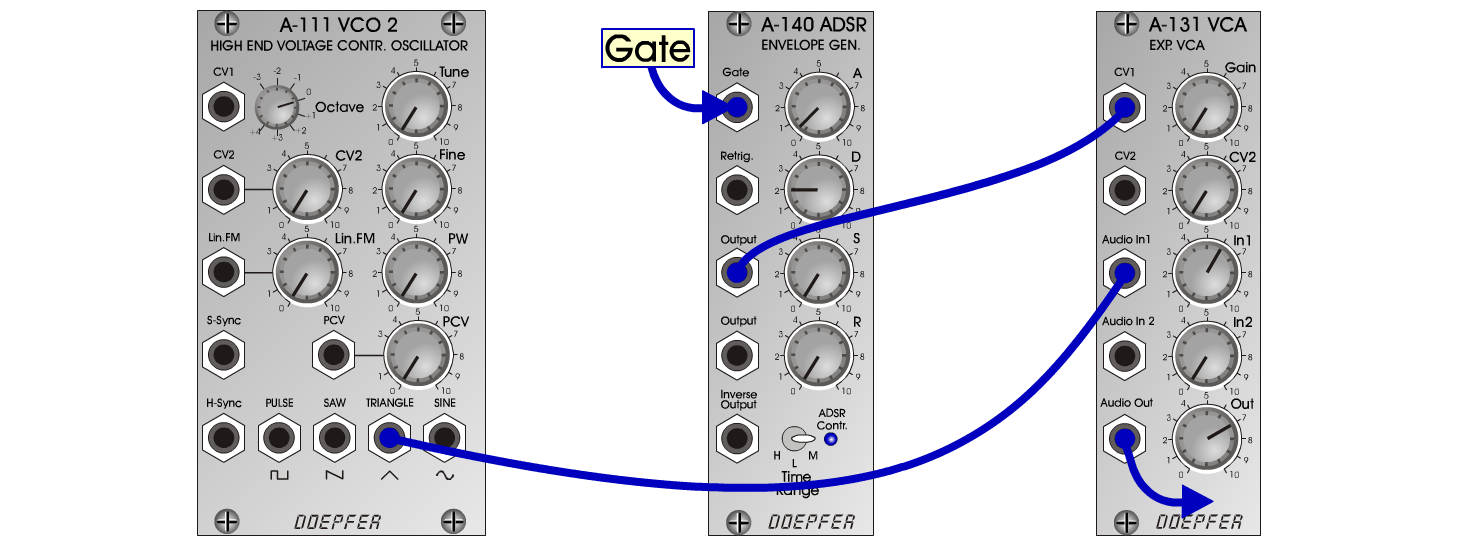TristarS y n t h> D o e p f e r A - 100 Connections: Settings: Tristar1 A-111 (Tri) <=> A-131 (Audio In)   A-140 (Output) <=> A-131 (CV1)   A-131 (Audio Out) <=> Amplifier A-140/1 (A = 0.5, D = 2, S = 0, R = 0, Range = M)   A-131 (Gain = 0, Audio In 1 = 6, Audio Out = 7) Tristar2 A-140/1 (A = 1.5, D = 2, S = 2, R = 5, Range = M) Tristar3 A-140/1 (A = 1, D = 6, S = 0, R = 5, Range = M Tristar4 A-140/1 (A = 1, D = 7.5, S = 0, R = 0, Range = M) Tristar5 A-140/1 (A = 5, D = 8, S = 6, R = 5, Range = M) A-140/1 (A = 5, D = 8, S = 6, R = 5, Range = M) Josef MuellerSound samples Tristar 1 (65 Herz) Tristar 1 (131 Herz) Tristar 1 (262 Herz) Tristar 2 (65 Herz) Tristar 2 (131 Herz) Tristar 2 (262 Herz) Tristar 3 (65 Herz) Tristar 3 (131 Herz) Tristar 3 (262 Herz) Tristar 4 (65 Herz) Tristar 4 (131 Herz) Tristar 4 (262 Herz) Tristar 5 (65 Herz) Tristar 5 (131 Herz) Tristar 5 (262 Herz)
br>